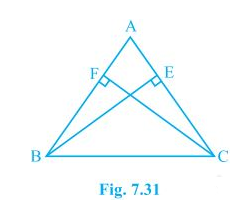# ABC is an isosceles triangle in which altitudes BE and CF are drawn to equal sides AC and AB respectively (see Fig.) . Show that these altitudes are equal.#### Solution

Given

AB=AC,

BE and CF are altitudes

To Prove

BE=CF

Proof

From ∆AEB and ∆AFC,

∠E=∠F

∠A=∠A

AB=AC

∴∆AEB≅∆AFC [A.A.S congruency]

BE=CF(18)(2)